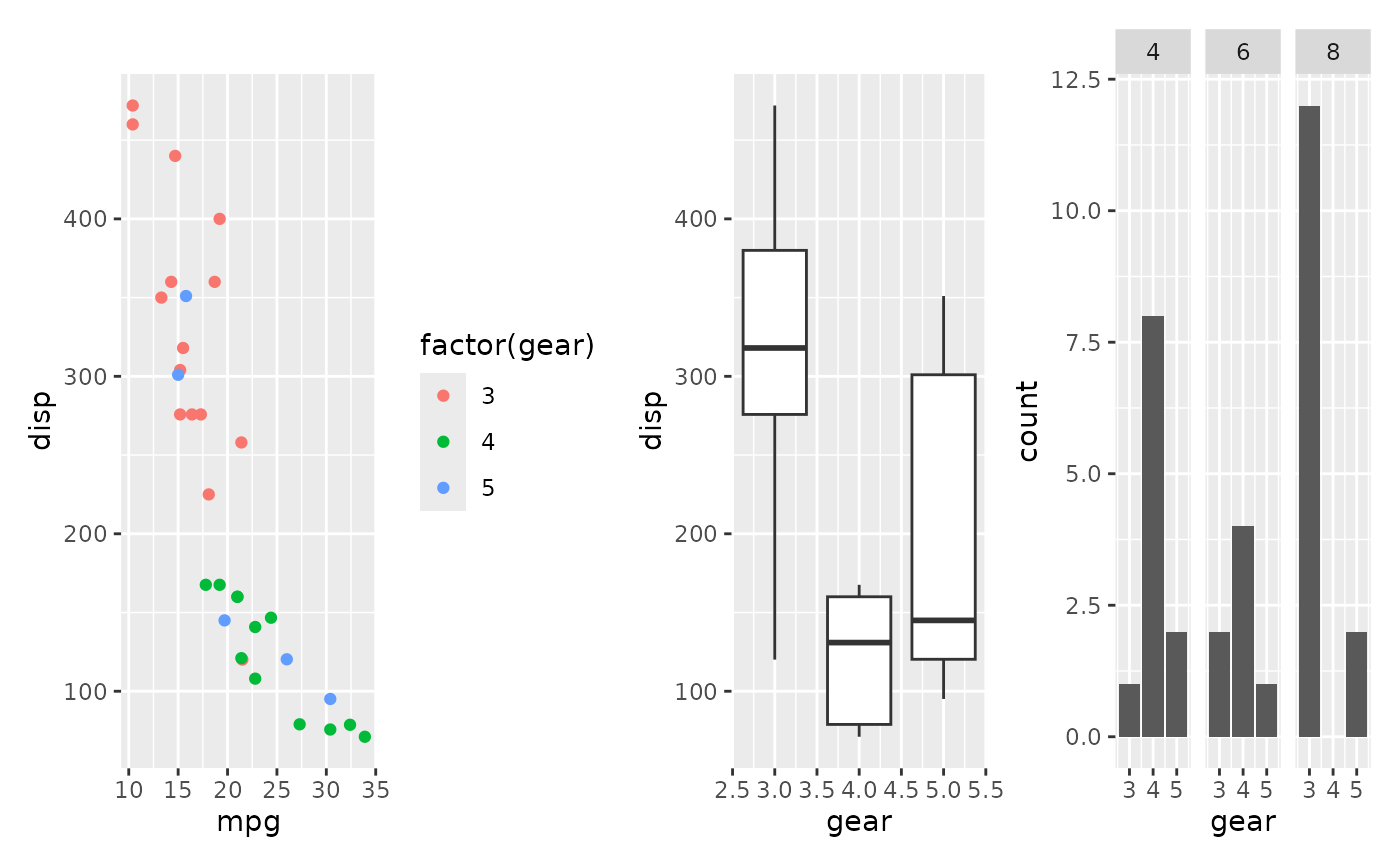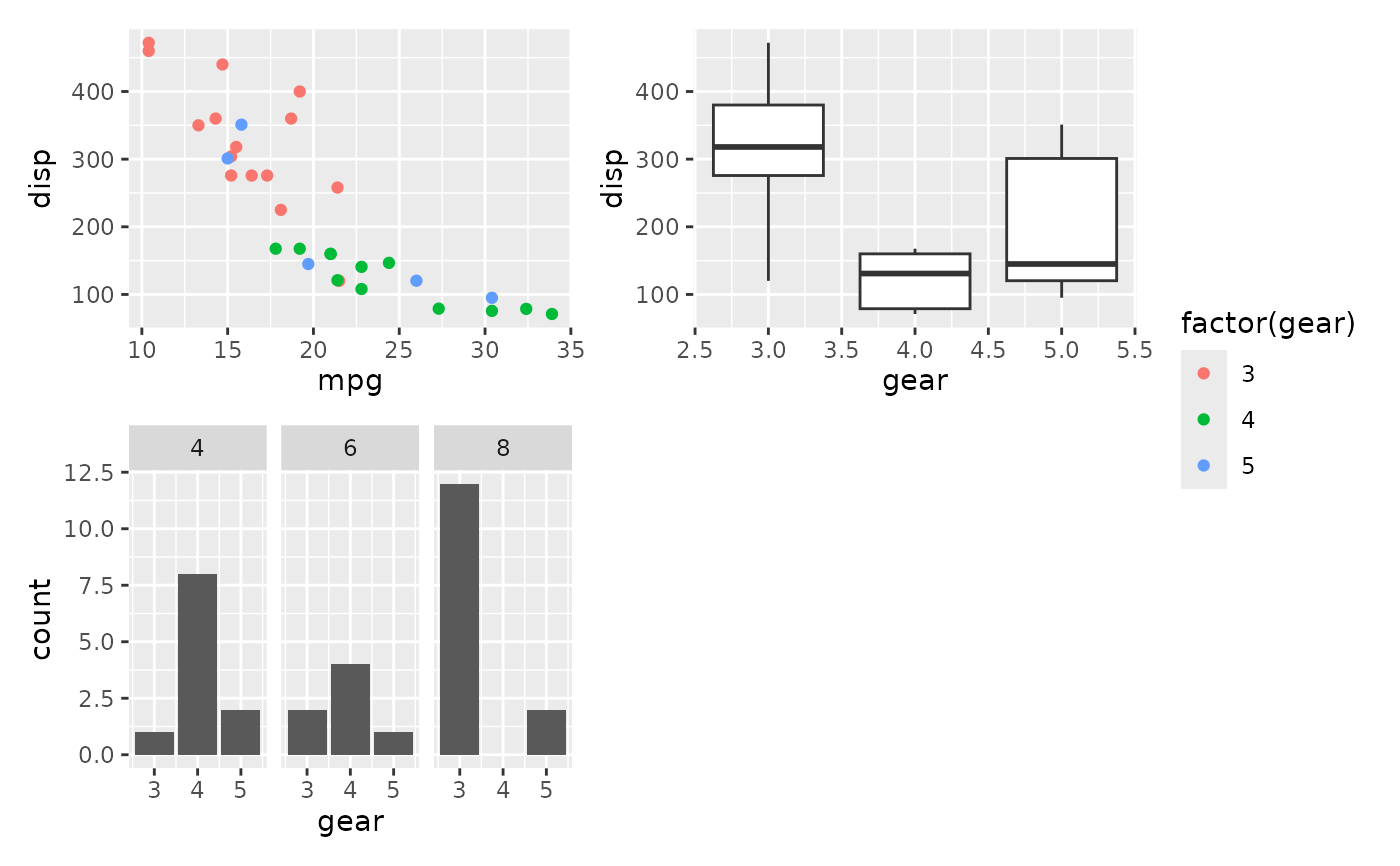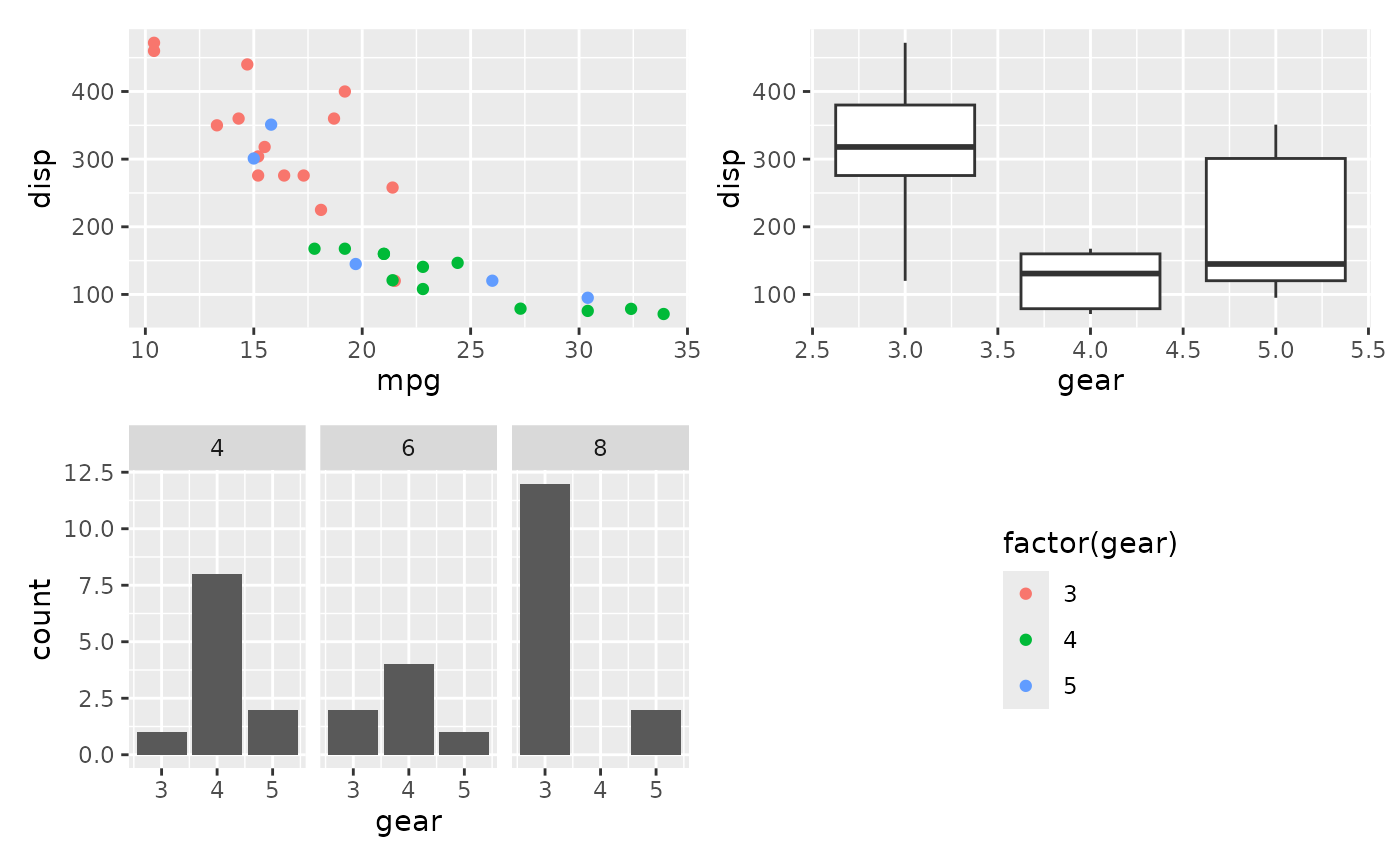Using the guides argument in plot_layout() you can collect and collapse guides from plots. By default these guides will be put on the side like with regular plots, but by adding a guide_area() to the plot you can tell patchwork to place the guides in that area instead. If guides are not collected or no guides exists to collect it behaves as a standard plot_spacer() instead.

guide_area()

Examples

library(ggplot2) p1 <- ggplot(mtcars) + geom_point(aes(mpg, disp, colour = factor(gear))) p2 <- ggplot(mtcars) + geom_boxplot(aes(gear, disp, group = gear)) p3 <- ggplot(mtcars) + geom_bar(aes(gear)) + facet_wrap(~cyl) # Guides are by default kept beeside their plot p1 + p2 + p3# They can be collected and placed on the side (according to the patchwork # theme) p1 + p2 + p3 + plot_layout(guides = 'collect', ncol = 2)# Using guide_area() you can also designate an empty area for this p1 + p2 + p3 + guide_area() + plot_layout(guides = 'collect')